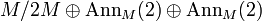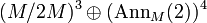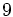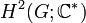# Difference between revisions of "Group cohomology of dihedral group:D8"

View group cohomology of particular groups | View other specific information about dihedral group:D8

## Family contexts

Family name Parameter value Information on group cohomology of family
dihedral group$D_{2n}$ of degree$n$, order$2n$ degree$n = 4$, order$2n = 8$ group cohomology of dihedral groups

## Homology groups for trivial group action

FACTS TO CHECK AGAINST (homology group for trivial group action):
First homology group: first homology group for trivial group action equals tensor product with abelianization
Second homology group: formula for second homology group for trivial group action in terms of Schur multiplier and abelianization|Hopf's formula for Schur multiplier
General: universal coefficients theorem for group homology|homology group for trivial group action commutes with direct product in second coordinate|Kunneth formula for group homology

### Over the integers

The homology groups over the integers are given as follows:$H_q(D_8;\mathbb{Z}) = \left \lbrace \begin{array}{rl} \mathbb{Z}, & q = 0 \\(\mathbb{Z}/2\mathbb{Z})^{(q + 3)/2}, & q \equiv 1 \pmod 4\\ (\mathbb{Z}/2\mathbb{Z})^{(q + 1)/2} \oplus \mathbb{Z}/4\mathbb{Z}, & q \equiv 3 \pmod 4 \\(\mathbb{Z}/2\mathbb{Z})^{q/2}, & q \mbox{ even }, q > 0 \\ \end{array}\right.$

The first few homology groups are given below:$q$$0$$1$$2$$3$$4$$5$$6$$7$$8$$H_q$$\mathbb{Z}$$\mathbb{Z}/2\mathbb{Z} \oplus \mathbb{Z}/2\mathbb{Z}$$\mathbb{Z}/2\mathbb{Z}$$\mathbb{Z}/2\mathbb{Z} \oplus \mathbb{Z}/2\mathbb{Z} \oplus \mathbb{Z}/4\mathbb{Z}$$\mathbb{Z}/2\mathbb{Z} \oplus \mathbb{Z}/2\mathbb{Z}$$(\mathbb{Z}/2\mathbb{Z})^4$$(\mathbb{Z}/2\mathbb{Z})^3$$(\mathbb{Z}/2\mathbb{Z})^4 \oplus \mathbb{Z}/4\mathbb{Z}$$(\mathbb{Z}/2\mathbb{Z})^4$

### Over an abelian group

The homology groups over an abelian group$M$ are given as follows:$H_q(D_8;M) = \left \lbrace \begin{array}{rl} M, & q = 0 \\(M/2M)^{(q + 3)/2} \oplus (\operatorname{Ann}_M(2))^{(q - 1)/2}, & q \equiv 1 \pmod 4\\ (M/2M)^{q/2} \oplus (\operatorname{Ann}_M(2))^{(q + 2)/2}, & q \equiv 2 \pmod 4 \\(M/2M)^{(q + 1)/2} \oplus M/4M \oplus (\operatorname{Ann}_M(2))^{(q - 1)/2}, & q \equiv 3 \pmod 4 \\(M/2M)^{q/2} \oplus (\operatorname{Ann}_M(2))^{q/2} \oplus \operatorname{Ann}_M(4), & q \equiv 0 \pmod 4, q > 0 \\ \end{array}\right.$ The first few homology groups with coefficients in an abelian group$M$ are given below:$q$$0$$1$$2$$3$$4$$5$$6$$7$$8$$H_q$$M$$M/2M \oplus M/2M$$M/2M \oplus \operatorname{Ann}_M(2) \oplus \operatorname{Ann}_M(2)$$(M/2M)^2 \oplus M/4M \oplus \operatorname{Ann}_M(2)$$(M/2M)^2 \oplus (\operatorname{Ann}_M(2))^2 \oplus \operatorname{Ann}_M(4)$$(M/2M)^4 \oplus (\operatorname{Ann}_M(2))^2$$(M/2M)^3 \oplus (\operatorname{Ann}_M(2))^4$$(M/2M)^4 \oplus M/4M \oplus (\operatorname{Ann}_M(2))^3$$(M/2M)^4 \oplus (\operatorname{Ann}_M(2))^4 \oplus \operatorname{Ann}_M(4)$

## Cohomology groups for trivial group action

FACTS TO CHECK AGAINST (cohomology group for trivial group action):
First cohomology group: first cohomology group for trivial group action is naturally isomorphic to group of homomorphisms
Second cohomology group: formula for second cohomology group for trivial group action in terms of Schur multiplier and abelianization
In general: dual universal coefficients theorem for group cohomology relating cohomology with arbitrary coefficientsto homology with coefficients in the integers. |Cohomology group for trivial group action commutes with direct product in second coordinate | Kunneth formula for group cohomology

### Over the integers

The cohomology groups over the integers are given as follows:$H^q(D_8;\mathbb{Z}) = \left \lbrace \begin{array}{rl} \mathbb{Z}, & q =0 \\(\mathbb{Z}/2\mathbb{Z})^{(q - 1)/2}, & q \equiv 1 \pmod 4\\ (\mathbb{Z}/2\mathbb{Z})^{(q/2} \oplus \mathbb{Z}/4\mathbb{Z}, & q \equiv 0 \pmod 4, q \ne 0\\(\mathbb{Z}/2\mathbb{Z})^{(q+2)/2}, & q \equiv 2 \pmod 4 \\ \end{array}\right.$

The first few cohomology groups are given below:$q$$0$$1$$2$$3$$4$$5$$6$$7$$8$$9$$H^q$$\mathbb{Z}$ 0$\mathbb{Z}/2\mathbb{Z} \oplus \mathbb{Z}/2\mathbb{Z}$$\mathbb{Z}/2\mathbb{Z}$$\mathbb{Z}/2\mathbb{Z} \oplus \mathbb{Z}/2\mathbb{Z} \oplus \mathbb{Z}/4\mathbb{Z}$$\mathbb{Z}/2\mathbb{Z} \oplus \mathbb{Z}/2\mathbb{Z}$$(\mathbb{Z}/2\mathbb{Z})^4$$(\mathbb{Z}/2\mathbb{Z})^3$$(\mathbb{Z}/2\mathbb{Z})^4 \oplus \mathbb{Z}/4\mathbb{Z}$$(\mathbb{Z}/2\mathbb{Z})^4$

### Over an abelian group

The cohomology groups over an abelian group$M$ are given as follows:$H^q(D_8;M) = \left \lbrace \begin{array}{rl} M, & q = 0 \\(\operatorname{Ann}_M(2))^{(q + 3)/2} \oplus (M/2M)^{(q - 1)/2}, & q \equiv 1 \pmod 4\\ (\operatorname{Ann}_M(2))^{q/2} \oplus (M/2M)^{(q + 2)/2}, & q \equiv 2 \pmod 4 \\(\operatorname{Ann}_M(2))^{(q + 1)/2} \oplus \operatorname{Ann}_M(4) \oplus (M/2M)^{(q - 1)/2}, & q \equiv 3 \pmod 4 \\(\operatorname{Ann}_M(2))^{q/2} \oplus (M/2M)^{q/2} \oplus M/4M, & q \equiv 0 \pmod 4, q > 0 \\ \end{array}\right.$

The first few cohomology groups with coefficients in an abelian group$M$ are:$q$$0$$1$$2$$3$$4$$5$$6$$7$$H^q$$M$$\operatorname{Ann}_M(2) \oplus \operatorname{Ann}_M(2)$$M/2M \oplus M/2M \oplus \operatorname{Ann}_M(2)$$M/2M \oplus \operatorname{Ann}_M(2) \oplus \operatorname{Ann}_M(2) \oplus \operatorname{Ann}_M(4)$$(M/2M)^2 \oplus M/4M \oplus (\operatorname{Ann}_M(2))^2$$(M/2M)^2 \oplus (\operatorname{Ann}_M(2))^4$$(M/2M)^4 \oplus (\operatorname{Ann}_M(2))^3$$(M/2M)^3 \oplus (\operatorname{Ann}_M(2))^4 \oplus \operatorname{Ann}_M(4)$

## Second cohomology groups and extensions

### Schur multiplier

The Schur multiplier, defined as second cohomology group for trivial group action$H^2(G;\mathbb{C}^\ast)$, and also as the second homology group$H_2(G;\mathbb{Z})$, is cyclic group:Z2.

This has implications for projective representation theory of dihedral group:D8.

### Schur covering groups

The three possible Schur covering groups for dihedral group:D8 are: dihedral group:D16, semidihedral group:SD16, and generalized quaternion group:Q16. For more, see second cohomology group for trivial group action of D8 on Z2, where these correspond precisely to the stem extensions.

### Second cohomology groups for trivial group action

Group acted upon Order Second part of GAP ID Second cohomology group for trivial group action (as an abstract group) Order of second cohomology group Extensions Number of extensions up to pseudo-congruence, i.e., number or orbits under automorphism group actions Cohomology information
cyclic group:Z2 2 1 elementary abelian group:E8 8 direct product of D8 and Z2, SmallGroup(16,3), nontrivial semidirect product of Z4 and Z4, dihedral group:D16, semidihedral group:SD16, generalized quaternion group:Q16 6 second cohomology group for trivial group action of D8 on Z2
cyclic group:Z4 4 1 elementary abelian group:E8 8 direct product of D8 and Z4, nontrivial semidirect product of Z4 and Z8, SmallGroup(32,5), central product of D16 and Z4, SmallGroup(32,15), wreath product of Z4 and Z2 6 second cohomology group for trivial group action of D8 on Z4
Klein four-group 4 2 elementary abelian group:E64 64 [SHOW MORE] 11 second cohomology group for trivial group action of D8 on V4

### Baer invariants

Subvariety of the variety of groups General name of Baer invariant Value of Baer invariant for this group
abelian groups Schur multiplier cyclic group:Z2
groups of nilpotency class at most two 2-nilpotent multiplier
groups of nilpotency class at most three 3-nilpotent multiplier
any variety of groups containing all groups of nilpotency class at most three --

## GAP implementation

### Computation of integral homology

The homology groups for trivial group action with coefficients in$\mathbb{Z}$ can be computed in GAP using the GroupHomology function in the HAP package, which can be loaded by the command LoadPackage("hap"); if it is installed but not loaded. The function outputs the orders of cyclic groups for which the homology or cohomology group is the direct product of these (more technically, it outputs the elementary divisors for the homology or cohomology group that we are trying to compute).

Here are computations of the first few homology groups:

#### Computation of first homology group

gap> GroupHomology(DihedralGroup(8),1);
[ 2, 2 ]

The way this is to be interpreted is that the first homology group (the abelianization) is the direct sum of cyclic groups of the orders listed, so in this case we get that$H_1(D_8;\mathbb{Z})$ is$\mathbb{Z}/2\mathbb{Z} \oplus \mathbb{Z}/2\mathbb{Z}$, which is the Klein four-group.

#### Computation of second homology group

gap> GroupHomology(DihedralGroup(8),2);
[ 2 ]

This says that the second homology group (the Schur multiplier) is cyclic group:Z2.

#### Computation of first few homology groups

To compute the first eight homology groups, do:

gap> List([1,2,3,4,5,6,7,8],i->[i,GroupHomology(DihedralGroup(8),i)]);
[ [ 1, [ 2, 2 ] ], [ 2, [ 2 ] ], [ 3, [ 2, 2, 4 ] ], [ 4, [ 2, 2 ] ], [ 5, [ 2, 2, 2, 2 ] ], [ 6, [ 2, 2, 2 ] ], [ 7, [ 2, 2, 2, 2, 4 ] ], [ 8, [ 2, 2, 2, 2 ] ] ]